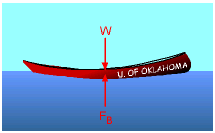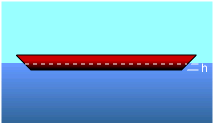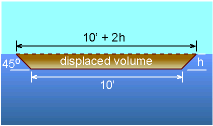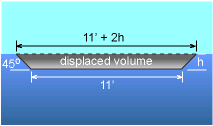Ch 2. Fluid Statics Multimedia Engineering Fluids PressureVariation PressureMeasurement HydrostaticForce(Plane) HydrostaticForce(Curved) Buoyancy
 Chapter 1. Basics 2. Fluid Statics 3. Kinematics 4. Laws (Integral) 5. Laws (Diff.) 6. Modeling/Similitude 7. Inviscid 8. Viscous 9. External Flow 10. Open-Channel Appendix Basic Math Units Basic Equations Water/Air Tables Sections Search eBooks Dynamics Fluids Math Mechanics Statics Thermodynamics Author(s): Chean Chin Ngo Kurt Gramoll ©Kurt GramollFLUID MECHANICS - CASE STUDY SOLUTIONForce EquilibriumSubmerged Water Line The total weight of the canoe and the paddlers is      W = 120 lb + 630 lb = 750 lb For equilibrium, the total weight must be balanced by the buoyant force. That is,      W = FB where the buoyant force is the weight of the liquid displaced by the volume and is given by      FB = ρgVdisplaced The submerged waterline is denoted as h and the displaced volume for each design will be expressed in terms of h as follows: For Design Layout A:Displaced Volume of Design Layout A The displaced volume of layout A is given by      Vdisplaced = 2 (10 + h) h Applying force equilibrium yields      750 = (1.94) (32.2) (2) (10 + h)h      h2 + 10h - 6 = 0 Using the Quadratic Equation gives,      h = 0.5678 ft The submerged height h (0.568 ft) is less than the height of the canoe (1 ft), hence this canoe will float. For Design Layout B:Displaced Volume of Design Layout B The displaced volume of layout B is given by      Vdisplaced = 2 (11 + h) h Applying force equilibrium yields      750 = (1.94) (32.2) (2) (11 + h)h      h2 + 11h - 6 = 0      h = 0.5208 ft The submerged height h (0.521 ft) is greater than the height of the canoe (0.5 ft), hence this canoe will sink.

Practice Homework and Test problems now available in the 'Eng Fluids' mobile app
Includes over 250 problems with complete detailed solutions.
Available now at the Google Play Store and Apple App Store.